The Theory of Special Relativity

In this paper, I will be describing the Theory of Special Relativity with simple, logical examples that require only simple math.  I am hoping to explain it in such a way that anyone reading this paper can learn, and more importantly, understand, the theory with as little effort as possible.

Before I get into what the consequences of Special Relativity are, I must first give some background information that led to the development of the theory.

In the way that sound travels through air at a constant speed, before Special Relativity was developed it was firmly believed that light travelled through a medium called the ether at a constant speed.  This ether was considered to be at absolute rest, and everything in the universe moved through the ether.  In 1887, Albert A. Michelson and Edward W. Morley performed what is now called the Michelson-Morley Experiment in which they tried to detect how fast and in what direction the Earth was moving through the ether.  Without going into the details of how the experiment was done, I'll just say that they could not detect the presence of the ether at all:  it appeared as though the earth was at rest with the ether.  The were many theories proposed to explain this unexpected result:  notable ones being ether drag, in which objects moving through the ether drag some of the ether along with it like when you run a knife through a viscous liquid, which would explain why the Earth did not seem to be moving with respect to the ether; and George F. Fitzgerald suggested that objects moving through the ether are contracted, or 'squished' in the direction of motion.  Ether drag was disproven by some stellar phenomenon, but there was no evidence against Fitzgerald's suggestion.

Eighteen years later, the Michelson-Morley Experiment was still being repeated by Morley and others.  People must have been convinced that the ether existed and thought that the experiment was flawed.  These experiments still gave the same results:  the Earth was not moving with respect to the ether.  Then, in 1905, an unknown patent office clerk named Albert Einstein proposed in a paper of his called "On the Electrodynamics of Moving Bodies" that

·         The laws of physics are the same in all inertial systems and that no preferred inertial system exists (he called this The Principle of Relativity), and that

·         The speed of light in a vacuum has the same value, c, in all inertial systems (The Principle of the Constancy of the Speed of Light).

Similar things had been suggested in other papers, but apparently Einstein was unaware of them. The Special Theory of Relativity is derived from these two assumptions.

First, I must explain what an inertial system, also called an inertial frame, is.  "Newton's First Law" ("The Law of Inertia"), states that an object at rest (not moving) will stay at rest unless acted upon by a force, and that an object in motion will stay in motion (at the same speed and direction of motion) unless acted upon by a force.  If you are in a train moving at a constant speed and direction, you are in an inertial frame.  Being in a car that is moving at a constant speed and direction is also an inertial frame.  There are also frames called non-inertial frames.  These are frames where objects are being accelerated, such as a car while it is accelerating.  Special Relativity is only capable of dealing with inertial frames.  General Relativity, which took Einstein ten years to develop after he developed Special Relativity, is used to deal with the things that go on while an object is under acceleration.

THE LORENTZ TRANSFORMATION

Einstein didn't start relativity from scratch.  In 1895, Hendrik Antoon Lorentz, in an effort to understand the apparent constancy of the speed of light, devised a mathematical translation used to translate (change) co-ordinates from one inertial frame to another.  His translation is called The Lorentz Translation.  To develop his translation, he started with the Galilean Transformation (x' = x - Vt;  y' = y;  z' = z;  t' = t.  See Figure. 1 at the end of this section for an explanation.  In the Galilean Transformation, time is constant, so time in one frame is the same as time in any other frame, so t' always would equal t), and tried to make x'² + y'² + z'² = ct'² (this is the formula for a point on a sphere that is expanding at the speed of light) translate to x² + y² + z² = ct² because for the 'stationary' frame, light is moving away from it at c, but the same light is moving away from the 'primed' frame (the one we are considering to be moving) at the same speed (because c is constant in all inertial frames), so the light should be at the same distance from both of them.  They should both be at the center of the sphere of light.  Using the Galilean Transformation, x'² + y'² + z'² = t'² would translate to - 2xVt + V²t² + y² + z² = c²t².  He had to find a new translation for t' = t that would cancel out the -2xVt and +V²t² terms.  Lorentz found that the correct translation is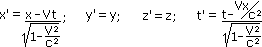(Notice that if V is is a lot less than the speed of light, it has almost no effect on the calculation)

Two variables were then created to make the formulas look simpler:

b(beta)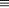v/c;  g (gamma)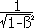(Notice that g will always be a number greater than 1 if V is not equal to zero)

Which made the x' and t' formulas

x' = g(x - bct); t' = g(t - bx/c)

and

x = g(x' + bct'); t = g(t' + bx'/c)

I am not sure if people paid much attention to Lorentz's transformation; most were probably still convinced that there was something wrong with the Michelson-Morley experiment or maybe ether drag had not been disproven when Lorentz derived his formulas.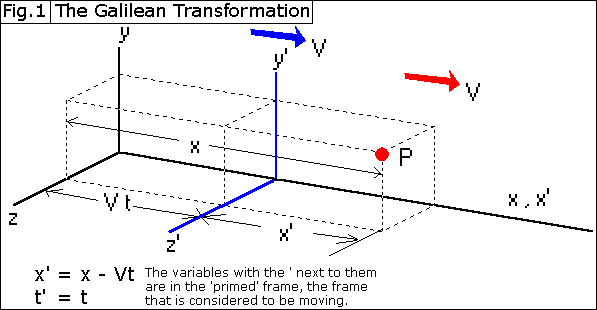Now on to the interesting stuff.

TIME DILATION

The first consequence of the assumption that the speed of light has the same value in any inertial frame is that time in different frames must pass at different rates.  To an observer, time in frames moving relative to his own frame appears to be passing at a slower rate than in his own.

Here is an example of why the rate that time passes at will be different in different frames.

Ignore the math on the right until you have read the explanation below the picture.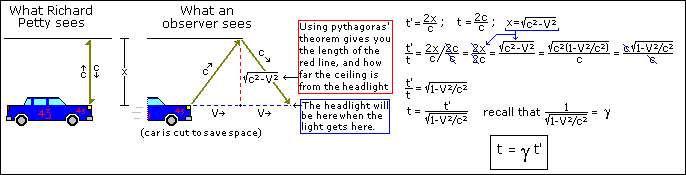Mr. Petty is racing through a tunnel.  His headlights are pointing straight up (he was in a crash earlier).  To Mr. Petty, the light from his headlight goes straight up at a speed of c, hits the ceiling, then returns to the headlight at a speed of c.  Since time is distance divided by velocity, the total time that passes from when the light leaves the headlight and returns is t'=2x/c.  Since we are considering Mr. Petty to be moving, he is in the 'primed' frame, which is why his t has an apostrophe next to it.

To the observer on the side of the track, whose frame we are considering to be stationary, the light moves along with the car, going at a speed of c in a diagonal direction relative to the observer (the light is always right above the headlight).

We then take a ratio of t' to t (t' / t ) and then substitute their values in.  With some simple grade 10 math and some substituting, we end up with t = gt'.  Since g is always greater than 1 if V is not zero, time in the primed frame passes at a slower rate than time in the stationary frame!  You may have noted that the time dilation formula looks different than Lorentz's time translation, t = g(t' + bx'/c).  This is because when we are measuring time, there is no value for x', so the +bx'/c term is equal to 0.

There is a slight contradiction here:  if there is no preferred inertial frame, then the 'moving' frame (Richard Petty) would see time going slower in the 'stationary' frame (the observer's).  The problem is that Special Relativity does not apply to non-inertial frames.  When an object is accelerating, we need to use General Relativity to understand what is going on.  The way I see it, the object that actually gains kinetic energy is the object that the observed effects of Special Relativity are actually happening to:  when a rocket burns fuel and gains kinetic energy, even though from the rocket, time in other frames seems to be going slower, it is in fact time in the rocket that is slowed, not time in the other frames.

Time Dilation has been proven numerous times in many different experiments.  Particles called pions, which 'live' for only 26ns before decaying into muons, are found to live many times longer if they are moving at very high speeds.  Also, highly accurate clocks have been put at the top and bottom of towers and it is found that the clock at the top of the tower runs slower than the one at the bottom because the clock at the top moves farther than the other one in the same amount of time when the Earth rotates.

An interesting thing to note about time dilation is that if you were going the speed of light, time around you would not pass, and if you were to go faster than the speed of light, you would arrive at your destination before you even started your journey!  However, it is impossible to go the speed of light, and just as impossible to go faster than the speed of light, as other parts of the theory show.

THE FITZGERALD-LORENTZ CONTRACTION

Fitzgerald was definitely on the right track when he suggested that objects contract in the direction of their movement.  Length contraction is closely related to time dilation:  the formula is the opposite of that for time dilation, and with t and t' switched to x and x':  the time dilation formula is t = gt', while the equation for length contraction that is observed from the stationary frame is x = x'/g.  That is, if someone in a 'stationary' frame takes a measurement of a length in a moving frame, he will get a smaller measurement than would someone who is in the moving frame because if g is greater than one, then the x measurement will be smaller than x' measurement.

For example, I have two 3m long boxes that each have a lamp at one end.  Light takes 10 nanoseconds to travel 3m, so if I turn on the lamp in one of the boxes, it will take 10ns for the light to hit the other side of the box.  I put one of the boxes on a rocket ship and now the box is moving at a velocity of 0.6c relative to me.  Both lamps turn on at the same time t=t'=0.  i observe the light to leave the lamp in each of the boxes at the same time, and the light hits the other end of the boxes at the same time in my frame.  In the box in my frame, the light takes 10ns to go from one side to the other.   Use the time dilation formula (t=gt') to find out how long the light takes to go from one end of the box in the moving frame to the other end.

t = 10ns;   V = 0.6c;   g = 1/sqrt(1 - v²/c²)

t = gt'

10ns = t' (1/sqrt(1 - v²/c²))

10ns = t' / sqrt(1 - (0.6c)²/c²)

10ns = t' / sqrt(1-0.36)

10ns = t' / sqrt(0.64)

10ns = t' / 0.8

t' = 8ns

So, if only 8ns has passed in the moving box and light went from one end to the other, the box can not be 3m long!  The moving box must only be 0.8 x 3m = 2.4m long!

Of course, a person in the box would still measure the box to be 3m long and he would measure the time to be 10ns.  The confusing thing here is that in Special Relativity, what is observed is not necessarily what has happened.  If I am stationary, and the two boxes are moving away from me at the same speed, but in opposite directions, then I would observe time to slow down the same rate in both of the boxes, and I would say that both boxes has contracted equally; however, someone in one of the boxes would look at the other box and say that time is going slower in that box than in his own box and the other box is also shorter than his box!  Again, this problem can only be solved using non-inertial frames and General Relativity.

Another problem that I had here that confused me was that though the Lorentz translations for x and t were nearly identical ( x = g(x' + bct'); t = g(t' + bx'/c) ), the equations I derived for x and t using these examples were opposites, dividing in one and multiplying in the other:  x = x'/g;  t = gt' !  What I didn't realize was that when I was measuring t, I was measuring something that is in my own frame, but my x was measuring the length of the box that is in the moving frame!  So x is actually a measurement of x' and x' is a measurement of x.

An interesting thing that I noted about this formula is that if you are going the speed of light, the term on the bottom of the equation, sqrt(1 - v²/c²), would give a zero, resulting in division by zero, which is an impossibility!  If the faster you go, the more contracted you got, you could extrapolate that if you managed to reach the speed of light, you would be contracted into nothingness!  This is just one of the formulas of Special Relativity that shows that it is impossible to reach the speed of light.

VELOCITY TRANSLATION

I often used to wonder why it was not possible to get something going the speed of light by firing it from an object that was already moving at a very high speed.  The reason is that if space is contracted in the moving frame, then if you fired something from that frame at a certain velocity, it would not be going as fast relative to the stationary frame!  The formula for translating velocities that are relative a frame that is considered to be moving is

Vo = (V + v')  /  (1 + Vv'/c²)

Vo is the velocity relative the stationary frame of the object being fired from an already moving frame, V is the velocity of the moving frame relative the stationary frame, and v' is the velocity of the object in question in the primed frame.

This formula also makes reaching the speed of light an impossiblity:  if V or V' is less than c, then Vo will be less than c, and if one of the velocities is c, no matter how high the other velocity is, Vo will be only c.

MASS INCREASE WITH VELOCITY

Special Relativity predicted that the faster an object moves, the more its mass will be.  Two formulas are needed to explain why this happens:  the first is the Velocity Translation (Vo = (v' + V)  /

(1 + v'V/c²)) and the second is

P = mV

This is the formula for force (P = force).  The faster on object is moving, the more force it will have; also the heavier an object is, the more force it will have when moving.For this example, we have 2 velcro-balls.  One is blue, the other red.  Both balls weigh the same thing when at rest (mo1 = mo2.  The o denotes that the mass is measured when the object is at rest).  When these balls collide, they stick together.

In the moving frame, the balls are moving toward each other each with the same velocity, v' = 0.5c.  When they collide in the moving frame, their forces are equal (because both their velocities are equal, and even though velocity does affect their masses, since the velocities are the same, their masses would still be the same), so they stick together and stop moving relative to the moving frame.  The moving frame, however, is moving away from the stationary frame at 0.5c, which means the balls are moving at 0.5c in relation to the stationary frame, too.

Now we will look at this collision from the view point of someone in the stationary frame.

Use the velocity translation to translate their velocities from the moving frame to the stationary frame.  We find that the red ball is moving at

Vo = (V + v')  /  (1 + Vv'/c²)

Vo = (0.5c + 0.5c)  /  (1 + (0.5c)(0.5c)/c²)

Vo = (c)  /  (1 + 0.25c²/c²)

Vo = 0.8c

and that the blue ball is moving at

Vo = (0.5c - 0.5c)  /  (1 + (0.5c)(0.5c)/c²)

Vo = (0.0c)  /  (1 + (0.25c²/c²)

Vo = 0.0c

So, in the stationary frame, the red ball has a force of

Pred = mv

Pred = m1 0.8c

and the blue one has a force of

Pblue = mv

Pblue = m2 0.0c

The balls collide and stick together.  Their collective mass is now M = m1 + m2.  Since the blue ball is not moving, it's mass is its rest mass, mo., so the total mass becomes m1 + mo.  Their total force is now P = Pblue + Pred = m1 0.8c + m2 0.0c = m1 0.8c.  Using the force equation, we can now solve for the mass of the red ball (the blue ball is at rest relative to our frame, so we know that it's mass has not changed).

P=MV

m1 0.8c = m1 + mo(0.5c)

m1 1.6 = m1 + mo

m1 1.6 - m1= mo

m1 0.6 = mo

m1 = mo/0.6 !

Remembering that the original velocity of m1 in the stationary frame was 0.8c, if we put 0.8c into g, we get 1/0.6, so the formula for the mass of a moving object is m = gmo, since mo/0.6 is the same as mo(1/0.6).  Using different velocities, you would find that this is the proper formula.

It is not hard to verify mass increase experimentally.  One just takes a particle at rest, measures its mass, then fires it at a high velocity at a surface that records how much force the particle hit with.  If the force is greater than moV by the same amount predicted by the theory, then the theory is correct.  Indeed, this has been verified.

This is another equation that limits objects to speeds under the speed of light.  The faster that you go, the smaller the denominator of the equation becomes, and the higher the mass becomes, and since more massive objects require more energy accelerate them, eventually you would find that you needed an infinite amount of energy to make the leap to the speed of light.

THE EQUIVALENCE OF ENERGY AND MASS

The most famous equation in the world is E=mc².  There is a good reason that this is a famous equation:  it revolutionized the world by bringing about the idea of using small amounts of matter to create huge amounts of energy.  Since c² is a huge number (9.00 x 10^16 m²/s²!), this means that a very small amount of mass is actually a huge amount of energy.

In our last section, we proved that the faster and object moves, the more it weighs.  And what is velocity but a manifestation of energy?  It takes energy to speed something up.  When an object is accelerated, it gains kinetic energy in the form of movement, velocity.  It also gains mass proportionally to how much much velocity energy it has, as was shown in the previous section.

In the formula, we know what m is, it a measurement of mass in kilograms (kg), and we know that c is the distant, in metres, that light travels in a second.  So the unit for energy is mc² = kg m²/s².  The m in this unit is a measurement in metres, not of mass, in this case.  Using any old physics text book, you can find that this unit, kg m²/s², is called a joule (J).

Einstein is reported to have said that there is enough energy in one matchstick to melt all the snow in Switzerland.  Using the formula, we can figure out how much energy it suppsedly would take to melt Switzerland's snow.

A match weighs about 1.00g, which is 0.00100kg, or 1.00 x 10^-3kg.

E = mc²

E = 1.00 x 10^-3kg (3.00 x 10^8 m/s)²

E = 1.00 x 10^-3kg (9.00 x 10^16 m²/s²)

E = 9.00 x 10^13 kg m²/s²

E = 9.00 x 10^13 J

That is a lot of energy!

In fact, the average person puts out roughly 2 x 10^11 J of energy in their entire lifetime, and the amount of energy in that little matchstick is 450 times this amount!

CONCLUSION

After learning Special Relativity something becomes very apparent:  Einstein's genius was not necessarily in math, but in logic and imagination.  After all, there was no math in the theory that a grade 10 student could not do.  The hard part, the part that Einstein was able to do and no one else, was taking the results from one situation and applying them to a new, different situation.  Einstein was able to see things that no one else had ever thought to look for.  It is his type of thought that everyone should strive toward.